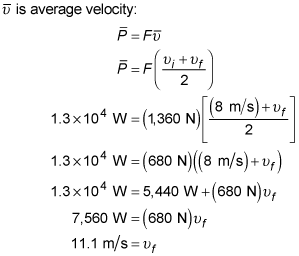##### Physics I: 501 Practice Problems For Dummies (+ Free Online Practice)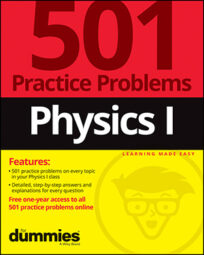Using physics, you can show the relationship between velocity and power. For example, you can calculate the velocity of a car using the velocity form of the power equation.

Here are some practice questions that you can try.

## Practice questions

1. The product of force and what quantity is equivalent to power?

2. At what velocity is a car moving at the instant its engine is using 2,000 watts to exert 100 newtons of force on the car's wheels?

3. Thirteen kilowatts of power are used to accelerate an 850-kilogram speedboat at a rate of 1.6 meters per second squared. If the boat's speed is 8 meters per second before the power kicks in, what is its velocity afterward?

Ignore friction and round your answer to the nearest tenth of a meter per second.

The following are the answers to the practice questions:

1. velocity

The basic definition of power is "the rate at which work is performed." Because work is the product of force and distance, the equation can be manipulated as follows: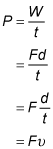where P is power, F is force, d is distance, t is time, and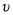is velocity. This shows that a secondary definition of power is the product of force exerted on an object and the object's acquired velocity.

2. 20 m/s

Use the velocity form of the power equation,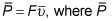is average power, F is force, and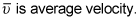For instantaneous situations — as in this problem — the average power is the power and the average velocity is the velocity: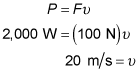3. 11.1 m/s

First convert the kilowatts of power into the "correct" units: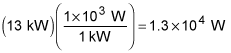Use the force form of Newton's second law to solve for the amount of force exerted on the speedboat: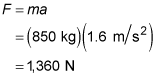Then use the velocity form of the power equation,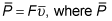is average power, F is force, and